Data Structures in Pandas

• Difficulty Level : Hard
• Last Updated : 18 Oct, 2021

Pandas is an open-source library that uses for working with relational or labeled data both easily and intuitively. It provides various data structures and operations for manipulating numerical data and time series. It offers a tool for cleaning and processes your data. It is the most popular Python library that is used for data analysis. In this article, We are going to learn about Pandas Data structure.

It supports two data structures:

Series

Pandas is a one-dimensional labeled array and capable of holding data of any type (integer, string, float, python objects, etc.)

Syntax: pandas.Series(data=None, index=None, dtype=None, name=None, copy=False, fastpath=False)

Parameters:

• data: array- Contains data stored in Series.
• index: array-like or Index (1d)
• dtype: str, numpy.dtype, or ExtensionDtype, optional
• name: str, optional
• copy: bool, default False

Example 1: Series holding the char data type.

Python3

 import pandas as pd  # a simple char listlist = ['g', 'e', 'e', 'k', 's']   # create series form a char listres = pd.Series(list)print(res)

Output: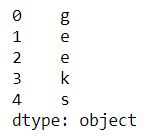Example 2: Series holding the Int data type.

Python3

 import pandas as pd  # a simple int listlist = [1,2,3,4,5]   # create series form a int listres = pd.Series(list)print(res)

Output: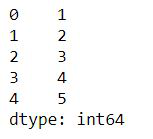Example 3: Series holding the dictionary.

Python3

 import pandas as pd dic = { 'Id': 1013, 'Name': 'MOhe',       'State': 'Maniput','Age': 24} res = pd.Series(dic)print(res)

Output: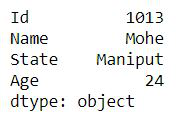Dataframe

Pandas DataFrame is a two-dimensional size-mutable, potentially heterogeneous tabular data structure with labeled axes (rows and columns). A Data frame is a two-dimensional data structure, i.e., data is aligned in a tabular fashion in rows and columns like a spreadsheet or SQL table, or a dict of Series objects. . Pandas DataFrame consists of three principal components, the data, rows, and columns.

Creating a Pandas DataFrame

In the real world, a Pandas DataFrame will be created by loading the datasets from existing storage, storage can be SQL Database, CSV file, and Excel file. Pandas DataFrame can be created from the lists, dictionary, and from a list of dictionary etc. Dataframe can be created in different ways here are some ways by which we create a dataframe:

Example 1: DataFrame can be created using a single list or a list of lists.

Python3

 # import pandas as pdimport pandas as pd  # list of stringslst = ['Geeks', 'For', 'Geeks', 'is',            'portal', 'for', 'Geeks']  # Calling DataFrame constructor on listdf = pd.DataFrame(lst)display(df)

Output: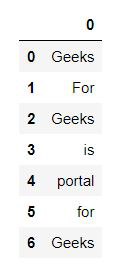Example 2: Creating DataFrame from dict of ndarray/lists.

To create DataFrame from dict of narray/list, all the narray must be of same length. If index is passed then the length index should be equal to the length of arrays. If no index is passed, then by default, index will be range(n) where n is the array length.

Python3

 # Python code demonstrate creating# DataFrame from dict narray / lists# By default addresses.  import pandas as pd  # initialise data of lists.data = {'Name':['Tom', 'nick', 'krish', 'jack'],        'Age':[20, 21, 19, 18]}  # Create DataFramedf = pd.DataFrame(data)  # Print the output.display(df)

Output: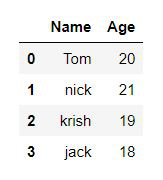Dealing with a column and row in DataFrame

Selection of column: In Order to select a column in Pandas DataFrame, we can either access the columns by calling them by their columns name.

Python3

 # Import pandas packageimport pandas as pd   # Define a dictionary containing employee datadata = {'Name':['Jai', 'Princi', 'Gaurav', 'Anuj'],        'Age':[27, 24, 22, 32],        'Address':['Delhi', 'Kanpur', 'Allahabad', 'Kannauj'],        'Qualification':['Msc', 'MA', 'MCA', 'Phd']}   # Convert the dictionary into DataFrame df = pd.DataFrame(data)   # select two columnsprint(df[['Name', 'Qualification']])

Output: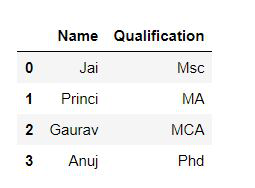How to Select Rows and Column from Pandas DataFrame?

Example 1: Selecting rows.

pandas.DataFrame.loc is a function used to select rows from Pandas DataFrame based on the condition provided.

Syntax: df.loc[df[‘cname’] ‘condition’]

Parameters:

• df: represents data frame
• cname: represents column name
• condition: represents condition on which rows has to be selected

Python3

 # Importing pandas as pdfrom pandas import DataFrame   # Creating a data frameData = {'Name': ['Mohe', 'Shyni', 'Parul', 'Sam'],        'ID': [12, 43, 54, 32],        'Place': ['Delhi', 'Kochi', 'Pune', 'Patna']       }   df = DataFrame(Data, columns = ['Name', 'ID', 'Place'])   # Print original data frameprint("Original data frame:\n")display(df)   # Selecting the product of Electronic Typeselect_prod = df.loc[df['Name'] == 'Mohe']   print("\n")   # Print selected rows based on the conditionprint("Selecting rows:\n")display (select_prod)

Output: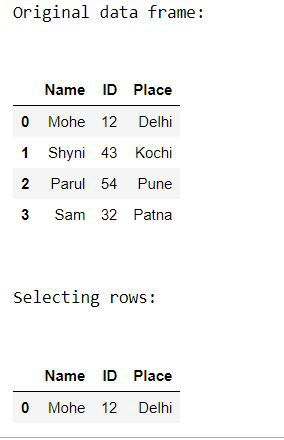Example 2: Selecting column.

Python3

 # Importing pandas as pdfrom pandas import DataFrame   # Creating a data frameData = {'Name': ['Mohe', 'Shyni', 'Parul', 'Sam'],        'ID': [12, 43, 54, 32],        'Place': ['Delhi', 'Kochi', 'Pune', 'Patna']       }   df = DataFrame(Data, columns = ['Name', 'ID', 'Place'])   # Print original data frameprint("Original data frame:")display(df)   print("Selected column: ")display(df[['Name', 'ID']] )

Output: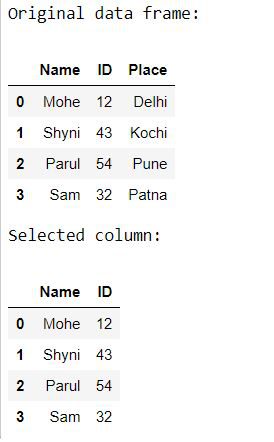My Personal Notes arrow_drop_up